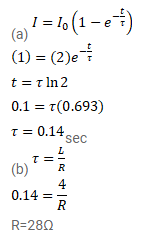# The current in a discharging LR circuit without the battery drops from

Question:

The current in a discharging LR circuit without the battery drops from $2.0 \mathrm{~A}$ to $1.0 \mathrm{~A}$ in $0.10 \mathrm{~s}$.

(a) Find the time constant of the circuit.

(b) If the inductance of the circuit is $4.0 \mathrm{H}$, what is its resistance?

Solution: